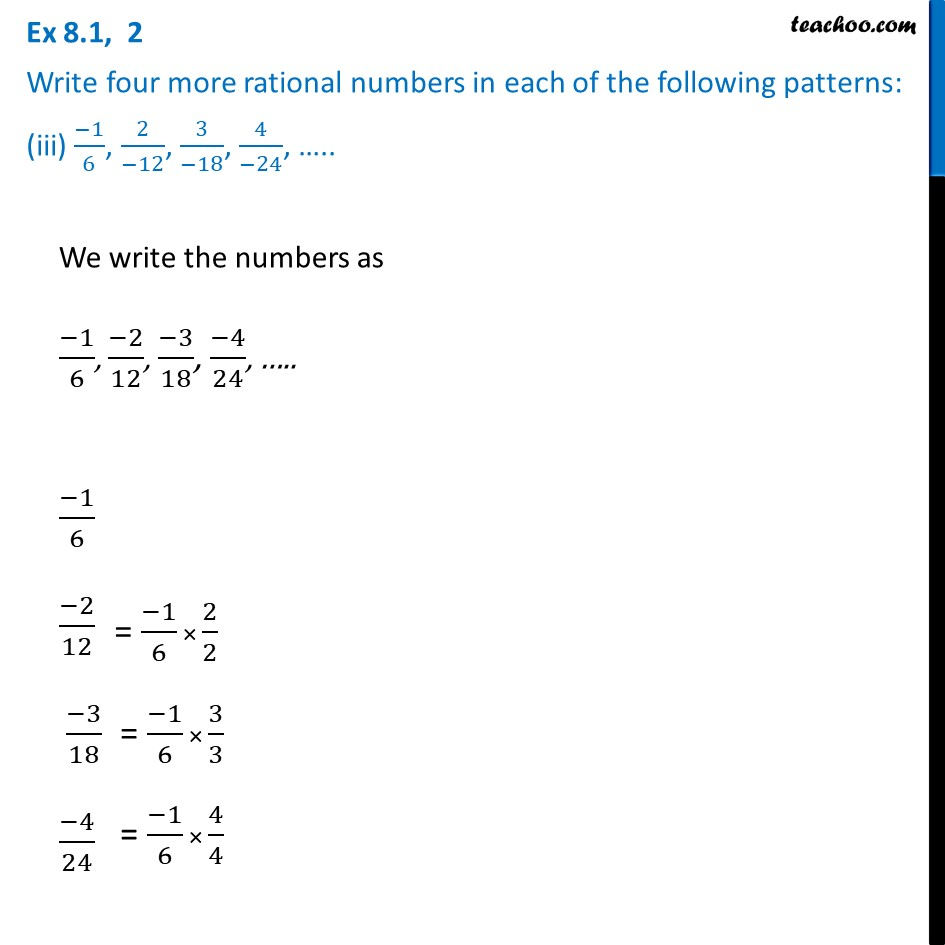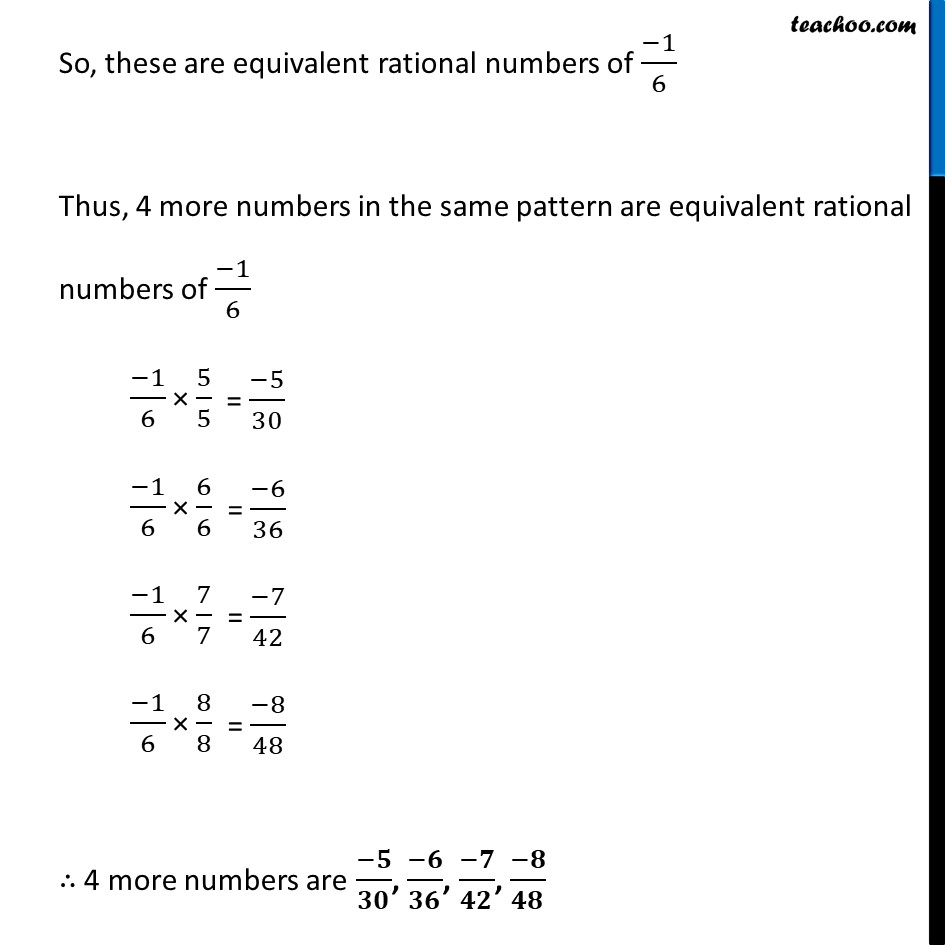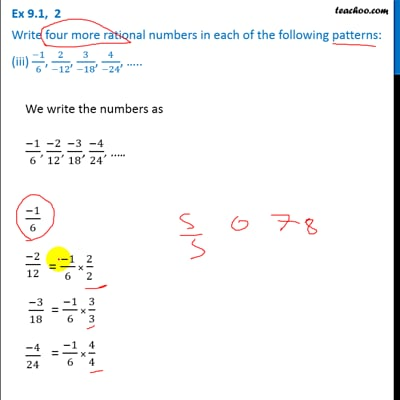Ex 8.1

Chapter 8 Class 7 Rational Numbers
Serial order wiseThis video is only available for Teachoo black users

Learn in your speed, with individual attention - Teachoo Maths 1-on-1 Class

### Transcript

Ex 8.1, 2 Write four more rational numbers in each of the following patterns: (iii) (−1)/6, 2/(−12), 3/(−18), 4/(−24), ….. We write the numbers as (−1)/6, (−2)/12, (−3)/18, (−4)/24, ….. (−1)/6 (−2)/12 (−3)/18 (−4)/24 So, these are equivalent rational numbers of (−1)/6 Thus, 4 more numbers in the same pattern are equivalent rational numbers of (−1)/6 (−1)/6 × 5/5 (−1)/6 × 6/6 (−1)/6 × 7/7 (−1)/6 × 8/8 ∴ 4 more numbers are (−𝟓)/𝟑𝟎, (−𝟔)/𝟑𝟔, (−𝟕)/𝟒𝟐, (−𝟖)/𝟒𝟖